# what is resistor

WHAT IS RESISTOR?

The meaning of the word resistor is opposition. Let us consider water tank as analogy.

Get More Electrical Concept And Interview Questions By Using This Link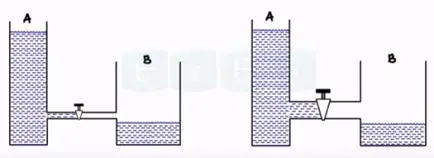We equate the pipe of the resistance and flow of water as flow of current. assume two tank A and B connected using two different pipes one as broad pipe and one as smaller pipe. Change in size of the pipe change in the intensity of the water flow larger pipe and small in the opposition hence water flow is more. Whereas as smaller the pipe water experience is more the resistance and flow reduces.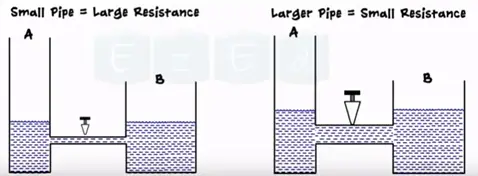In the same way the electronics a component that opposes the flow of current is called resistor. A symbol is the form of zigzag and its unit is ohms.Characteristics of Resistor

The characteristics of resistor are the resistance value power dissipation or power rating.

When an electrical current passes through the resistor electrical energy is lost by the resistor in form of heat. Greater the current flow the hotter the resistor will get this known as resistor power rating.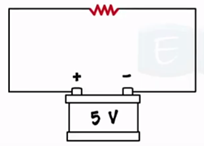Resistor is classified into two types. They are

• Fixed resistor
• Variable resistor

Fixed resistor is further classified into three types, they are

• Carbon composition resistor
• Wire wound resistor
• Metalized resistor

Fixed resistor

As per their name the value of the resistor is fixed. Example: 11Ω, 14 Ω 164 Ω etc.

Carbon composition resistor

It is most commonly used resistor. The resistor materials is carbon clay composition with plastic case coating surface. Hence it is called carbon composition resistor. leads are made up of tinned copper.

These resistors are cheap and reliable and have high stability.Wire wound Resistor

Wire wound resistor made by wounding of metal wire with nichrome around ceramic, plastic, fiber glass and of the wires are soldered or welded with two caps or rings attached to the ends of the core.

The assembly is protected with layer of paint by modulated plastic or enamel. Coating baked on high temperature.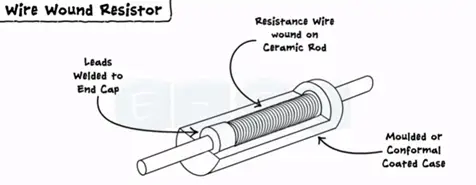Metalized resistor

It is a general purpose resistor. It is uses ceramic core coated with metal oxide. These resistors are electrically stable and reliable during high temperature operation.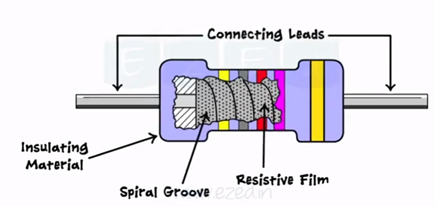Variable resistor

The very common implementation of resistor is volume control knob or regulator of fan.

The motion of the knob actually changes the resistance of the circuit and charges the level of the volume. Similarly the motion of the regulator charges the speed of the fan.

Every variable resistor has three leads one is variable and other two is fixed.

There are two types of variable resistor. They are

• Rheostat
• Potentiometer

Rheostat

It is two fixed leads and other one is variable and made one end to other end. This lead moves from left to right. Its resistance moves from minimum to maximum. The rheostat is used in the laboratories for experimental purpose.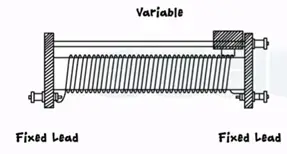Potentiometer

It has three leads A, B and W and center lead is adjustable lead varies from end A to B. here R at end A is minimum and b is at maximum.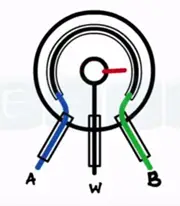In case of potentiometer also resistance varies in same manner as seen in rheostat mix resistance to maximum resistance. The practical potentiometer as shown the most common example of potentiometer is regulator of fan,

Colour coding technique of resistor

As a component resistor has different colour bands printed on resistor which decides the value.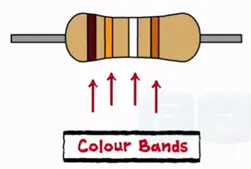How to calculate the value of resistor?

There are two types of resistors with four bands and five bands. Every colour band has its own decimal value which starts significance and it’s obtained from following table.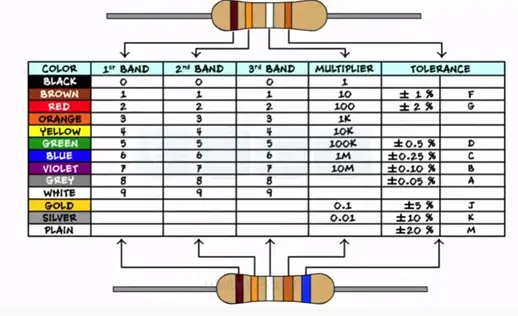To remember this table and value for the colour bands we follow the sentence.

“BB Roy of Great Britain has a Very Good Wife”

All capital letters represents the colours

Black Brown Red Orange Yellow Green Blue Violet Grey White

In case of four band resistor 1st,2nd of left determine the decimal value 3rd band is the multiplier 4th band is for tolerance. Consider R with four bands.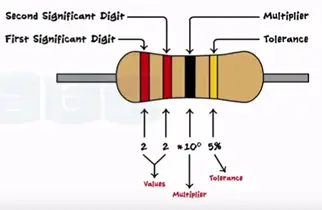Red, red, black and golden

From the tables we get the values 2 for left and black for 0 and 5% for golden

The total value of resistor is

22*10± 5%

22*1± 5%

22±5%Ω

Only modification in five band resistors is first three colour band determines the values and fourth band for multiplier and fifth band for tolerance.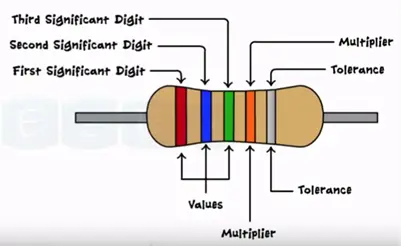Red blue green orange silver

265*10³ ±10%

265KΩ±10%